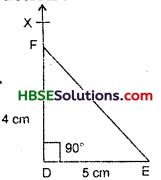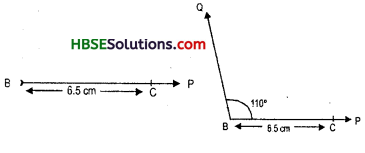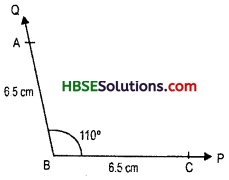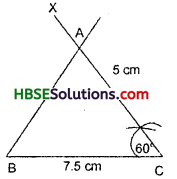# HBSE 7th Class Maths Solutions Chapter 10 Practical Geometry Ex 10.3

Haryana State Board HBSE 7th Class Maths Solutions Chapter 10 Practical Geometry Ex 10.3 Textbook Exercise Questions and Answers.

## Haryana Board 7th Class Maths Solutions Chapter 10 Practical Geometry  Exercise 10.3

Question 1.
Construct ADEF such that DE = 5 cm., DF = 4 cm and ∠EDF = 90°.
Solution:
Steps of Construction :
1. Draw DE = 5 cm.
2. At D draw ∠FDE = 90°.
3. Along DF, cut off DF = 4 cm.
4. Join E and F.
ΔDEF is the required triangle.Question 2.
Construct an isosecels triangle in which the length of each of its equal sides is 6.5 cm and the angle between them is 110°.
Solution:
Draw a rough sketch of ΔABC and indicate the lengths of its sides and the measure of the included angle.
Steps Of Construction :
1. Draw a ray BP.
2. From ray BP, cut off a line-segment BC = 6.5 cm. (Fig.)3. At B, construct ∠QBC = 110°. (Fig.)
4. From ray BQ, cut off a line-segment BA = 6.5 cm. (Fig.)5. Join AC to obtain the required triangle ABC. (Fig.)Question 3.
Construct ΔABC with BC = 7.5 cm, AC = 5 cm and m∠C = 60°.
Solution:
Steps of Construction :1. Draw a line segment BC = 7.5 cm.
2. At C, construct ∠BCX = 60°.
3. From the ray CX, cut off CA = 5 cm.
4. Join AB.
Thus, ABC is the required triangle.